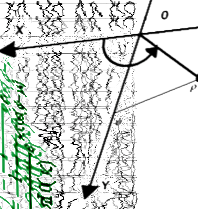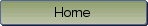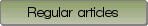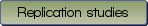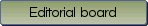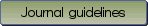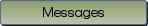Commentary on "A review of effect sizes and their confidence intervals, Part I: The Cohen's d family": The degrees of freedom for paired samples designs.

Bibliographic information: BibTEX format RIS format XML format APA style
Cited references information: BibTEX format APA style

Fitts, Douglas A.
281-294
Keywords: noncentral t distributions , parameter estimation , simulation
Tools: R, C
(no sample data)   (Appendix)

In their review of effect sizes of the Cohen's d family, Goulet-Pelletier and Cousineau (2018) proposed several methods for generating confidence intervals for the unbiased standardized mean difference, g. Among them they proposed using degrees of freedom \nu = 2(n - 1) instead of \nu = (n - 1) for all paired samples designs that use a pooled standard deviation to standardize the mean difference (pooled paired samples) when calculating g and its confidence limits from a noncentral t distribution. Simulations demonstrate that the exact \nu for a pooled paired samples design vary as a function of the population correlation \rho between 2(n - 1) at \rho = .0 and (n - 1) at \rho = 1.0. This affects the calculation of g and the selection of the appropriate noncentral t distribution for calculating the confidence limits. Using a sample r to estimate the unknown \rho causes a further deviation from the presumed noncentral t distribution even when the \nu are known. These facts adversely affect the coverage of the confidence intervals computed as recommended by the authors. These methods for calculating noncentral t confidence intervals should not be used as described with pooled paired samples designs. Noncentral confidence intervals for either a two sample design or a paired samples design where the mean difference is standardized by the standard deviation of the difference scores are unaffected by this problem. An R script and C source code are provided.

Be informed of the upcoming issues with RSS feed:RSS